# JP4727777B2 - Measuring method by scanning electron microscope - Google Patents

## Info

Publication number
JP4727777B2
JP4727777B2 JP14375399A JP14375399A JP4727777B2 JP 4727777 B2 JP4727777 B2 JP 4727777B2 JP 14375399 A JP14375399 A JP 14375399A JP 14375399 A JP14375399 A JP 14375399A JP 4727777 B2 JP4727777 B2 JP 4727777B2
Authority
JP
Japan
Prior art keywords
pattern
sample
length
length measurement
electron microscope
Prior art date
Legal status (The legal status is an assumption and is not a legal conclusion. Google has not performed a legal analysis and makes no representation as to the accuracy of the status listed.)
Expired - Fee Related
Application number
JP14375399A
Other languages
Japanese (ja)
Other versions
JP2000337846A (en
Inventor

Original Assignee

Priority date (The priority date is an assumption and is not a legal conclusion. Google has not performed a legal analysis and makes no representation as to the accuracy of the date listed.)
Filing date
Publication date
Application filed by 富士通セミコンダクター株式会社, 株式会社日立製作所 filed Critical 富士通セミコンダクター株式会社
Priority to JP14375399A priority Critical patent/JP4727777B2/en
Publication of JP2000337846A publication Critical patent/JP2000337846A/en
Application granted granted Critical
Publication of JP4727777B2 publication Critical patent/JP4727777B2/en
Anticipated expiration legal-status Critical
Application status is Expired - Fee Related legal-status Critical

## Images

•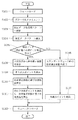•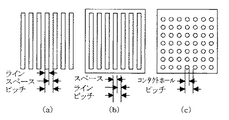•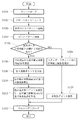•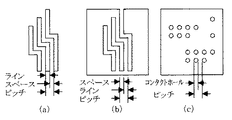•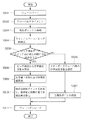••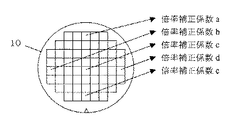•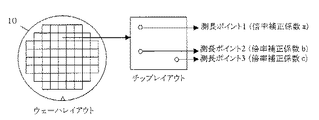•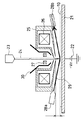••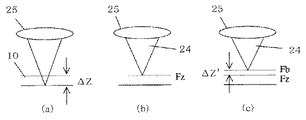•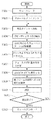•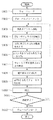•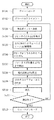•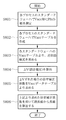•••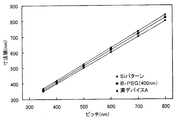## Classifications

• HELECTRICITY
• H01BASIC ELECTRIC ELEMENTS
• H01JELECTRIC DISCHARGE TUBES OR DISCHARGE LAMPS
• H01J37/00Discharge tubes with provision for introducing objects or material to be exposed to the discharge, e.g. for the purpose of examination or processing thereof
• H01J37/26Electron or ion microscopes; Electron or ion diffraction tubes
• H01J37/28Electron or ion microscopes; Electron or ion diffraction tubes with scanning beams
• HELECTRICITY
• H01BASIC ELECTRIC ELEMENTS
• H01JELECTRIC DISCHARGE TUBES OR DISCHARGE LAMPS
• H01J2237/00Discharge tubes exposing object to beam, e.g. for analysis treatment, etching, imaging
• H01J2237/26Electron or ion microscopes
• H01J2237/28Scanning microscopes
• H01J2237/2813Scanning microscopes characterised by the application
• H01J2237/2814Measurement of surface topography
• H01J2237/2816Length
• HELECTRICITY
• H01BASIC ELECTRIC ELEMENTS
• H01JELECTRIC DISCHARGE TUBES OR DISCHARGE LAMPS
• H01J2237/00Discharge tubes exposing object to beam, e.g. for analysis treatment, etching, imaging
• H01J2237/26Electron or ion microscopes
• H01J2237/282Determination of microscope properties
• H01J2237/2826Calibration

## Description


BACKGROUND OF THE INVENTION
The present invention relates to a length measuring method using a scanning electron microscope, and more particularly to a length measuring method using a scanning electron microscope suitable for length measurement and inspection of a semiconductor device.

[Prior art]
Recent scanning electron microscopes (SEMs), especially length measurement SEMs, have measured values that are true values, and the measured values have variations and offsets depending on the sample configuration, film type, etc. There is no need for it. Conventionally, as a dimension calibrating means of a length measurement SEM, a pitch pattern is created using a material (for example, Al, W, etc.) with less charge on Si, and a length measurement value is adjusted to the pitch pattern. The length measurement calibration value of the length measurement SEM was obtained.

Further, in recent measurement SEMs, the acceleration voltage of incident electrons on the primary side is increased to improve the resolution of secondary electrons, and a negative voltage is applied to the sample side to decelerate the acceleration voltage of incident electrons. An electric field method (hereinafter referred to as a retarding method) is employed. Specifically, in the retarding method, the acceleration voltage of electrons emitted from the electron gun portion is increased by + Vr, and a voltage of −Vr is applied to the sample, compared with the conventional method (sample-side voltage 0). The electrons emitted from the gun unit with an acceleration voltage of V + Vr are decelerated by the voltage of −Vr applied to the sample side after passing through the objective lens, and finally enter the sample with the acceleration voltage V. Has been. The characteristics of the retarding method are that the incident electrons on the primary side pass under the objective lens with the acceleration voltage V + Vr, so that the chromatic aberration of the lens can be reduced, and the secondary electrons generated from the sample are detected by the electric field of −Vr. The secondary electron collection efficiency is improved because the electron beam is accelerated in the direction of the vessel.

[Problems to be solved by the invention]
However, in the scanning electron microscope that employs the retarding method as described above, the acceleration voltage of the electron beam incident on the sample (hereinafter referred to as the “Landing Voltage”) varies depending on the voltage applied to the sample side. When the sample to be performed is covered with an insulating film, or when the insulating film is attached to the surface, the landing voltage changes and the magnification (Field of View) shifts. The dimension measurement of the length measurement SEM is performed under the condition that the Landing Voltage is constant and the magnification is constant. Therefore, when one of the values changes, there is a problem that the value of the measured dimension changes. That is, there is a problem that a dimensional error does not occur due to a change in the Landing Voltage, and the measured dimension is not a true value.

This problem also occurred in the conventional length measuring SEM that does not employ the retarding method. That is, the landing voltage is changed due to the change in the surface potential of the sample, resulting in a dimensional error and a problem that the measured dimension is not a true value.
Table 1 shows a Si pattern formed on a Si wafer by a conventional length measurement SEM, a 400 nm thick B-PSG film pattern, and a 10 nm thick SiO film. 2 On-film pattern, 100 nm thick SiO 2 It shows the result of measuring the pitch in the pattern on the film, the pattern on the SiN film, the pattern on the HTO + SiN film (35 nm), the pattern on the SiON film with a film thickness of 25 nm, and the pattern on the actual device A and the actual device B. . The measured pitch has a design value of 350 nm, 400 nm, 500 nm, 600 nm, 700 nm, and 800 nm. The unit of numerical values in the table is nm.

[Table 1]

FIG. 18 shows the pitch design value (horizontal axis) and SEM length measurement value (vertical) obtained from the data of the Si pattern, the B-PSG film pattern and the actual device A pattern among the length measurement data shown in Table 1. (Axis) is illustrated. Although the pitch measurement value of the Si pattern substantially matches the design value, the pitch measurement value of the pattern on the B-PSG insulating film and the pitch measurement value of the pattern on the actual device have a large deviation from the design value. I understand that. This is presumably because an error occurred in the measured value because the sample surface was charged up by electron beam irradiation during pattern length measurement on the B-PSG insulating film or the actual device.
The present invention has been made in view of such problems in length measurement using a conventional scanning electron microscope, and the measurement using a scanning electron microscope in which no length measurement error occurs due to the configuration of the sample or the film type. The purpose is to provide a long method.

[Means for Solving the Problems]
In order to solve the above problems, in the length measuring method using a scanning electron microscope of the present invention, a step of measuring a known pattern previously provided in a predetermined region on a sample by a scanning electron microscope, and the measurement is performed. Deriving the magnification correction coefficient by comparing the length result with the design value of the pattern, and multiplying the length measurement value of the measurement point measured by the scanning electron microscope by the magnification correction coefficient to obtain the true dimension. And obtaining a step.

In this method, for example, a sample provided with a specific test pattern such as a line-and-space or a contact hole for obtaining correction data in advance is used in a scribe in a semiconductor wafer sample or an empty area in a chip. Then, by measuring the test pattern and obtaining a magnification correction coefficient for correcting the measurement error, the dimension measurement error of the SEM caused by the charge-up of the sample surface (surface potential change at the location irradiated with the incident electrons) can be reduced. To compensate.

The length measuring method using a scanning electron microscope of the present invention also includes a step of measuring a pattern on a sample with a scanning electron microscope and comparing the length measurement result with a design value of the pattern to obtain a magnification correction coefficient. And a step of obtaining a true dimension by multiplying a length measurement value of a measurement point measured by a scanning electron microscope by the magnification correction coefficient. In this method, a dedicated test pattern for obtaining the magnification correction coefficient is not provided on the sample, and the sample surface is charged with a pattern that can measure the line width, space width, or pitch among the patterns of the actual sample. The magnification correction coefficient to be obtained is obtained.

The magnification correction coefficient may be derived for each sample. When the sample is a semiconductor wafer, a magnification correction coefficient may be derived for each chip including the measurement point. Further, the magnification correction coefficient may be derived using a pattern near the measurement point. The state of charge-up on the sample surface varies slightly depending on various factors such as the structure and material of the sample, or the state in which the sample is held on a stage or the like. Therefore, in order to compensate the measurement error of the SEM due to the charge-up with high accuracy, the pattern of the place as close as possible to the length measurement point is used, where the charge-up situation is considered to approximate the length measurement point. It is preferable to derive a magnification correction coefficient. When deriving the magnification correction coefficient for each chip, or when deriving the magnification correction coefficient using a pattern near the length measurement point, it is usually not a dedicated test pattern for obtaining the magnification correction coefficient, but an actual sample. The magnification correction coefficient is obtained using this pattern.
caused by.

In deriving the magnification correction coefficient, it is desirable to compare the length measurement result of the pattern pitch with the design value. This is because, in general, patterns formed by exposure to light may cause the line width and space width to deviate from the design values due to proximity effects, light interference, etc., but the pitch is roughly as designed. Because it becomes. When obtaining the pitch measurement results, it is desirable to average the measurement values of a plurality of pitches. One aspect of length measurement when using the length measurement result of the pitch to derive the magnification correction coefficient is to measure the pitch together with the line width and space width of the length measurement point in the SEM field of view. There is a method of correcting a length measurement value of a line or a space by a magnification correction coefficient derived from the result.

The length measuring method using the scanning electron microscope of the present invention also includes the step of autofocusing the scanning electron microscope to the measurement point using the output of the sensor for detecting the distance from the objective lens to the sample, and the excitation current of the objective lens. The step of detecting the excitation current ΔIobj of the objective lens corresponding to the focus shift by monitoring the change of the sample signal emitted from the sample while changing the value, and the acceleration voltage of the electron beam incident on the sample by the excitation current ΔIobj of the objective lens The method includes the step of converting to ΔV, the step of changing the acceleration voltage of the electron beam incident on the sample by ΔV, and the step of measuring the measurement point.

The autofocus may be performed by controlling the excitation current of the objective lens, or may be performed by mechanically moving a sample stage or the like on which the sample is placed. According to this method, the change in the electron beam acceleration voltage caused by the charge-up of the sample surface can be canceled and the acceleration voltage of the electron beam incident on the sample can be set as a set value. The error due to the charge-up is not included.

The length measuring method using the scanning electron microscope of the present invention also includes the step of autofocusing the scanning electron microscope to the measurement point using the output of the sensor that detects the distance from the objective lens to the sample, and the voltage applied to the sample. Monitoring the change in the sample signal emitted from the sample while changing the sample, detecting the sample applied voltage ΔVb corresponding to the focus shift, changing the electron beam acceleration voltage incident on the sample by ΔVb, and measurement points And a step of measuring the length.

The autofocus may be performed by controlling the excitation current of the objective lens, or may be performed by mechanically moving a sample stage or the like on which the sample is placed. According to this method, similarly to the above method, the change in the electron beam acceleration voltage caused by the charge-up of the sample surface can be canceled out, and the acceleration voltage of the electron beam incident on the sample can be set to the set value. The long value does not include an error due to charge-up of the sample surface.

The length measuring method using the scanning electron microscope according to the present invention includes a step of obtaining a correspondence relationship between an acceleration voltage of an electron beam incident on a sample and a magnification correction coefficient in advance, and an electron incident on a length measuring point on the sample. A step of obtaining an actual acceleration voltage of the beam, a step of obtaining a magnification correction coefficient corresponding to the actual acceleration voltage of the electron beam incident on the length measurement point with reference to the correspondence relationship obtained in advance, and a measurement point And a step of obtaining a true dimension by multiplying the length measurement value by the magnification correction coefficient.

In this method, the step of obtaining the actual acceleration voltage of the electron beam incident on the measurement point on the sample may be realized in any way, for example, the acceleration of the electron beam set in the scanning electron microscope A step of obtaining an objective lens correction current ΔIobj that eliminates a focus shift under an objective lens excitation condition corresponding to the voltage, and a step of converting the objective lens correction current ΔIobj into an acceleration voltage ΔV of an electron beam incident on the sample. be able to. Alternatively, it may be realized by obtaining the sample application voltage ΔVb that eliminates the focus shift under the objective lens excitation condition corresponding to the acceleration voltage of the electron beam set in the scanning electron microscope.

According to the length measurement method of the present invention, in the length measurement using the scanning electron microscope, it becomes possible to obtain a true dimension value by compensating for the length measurement error caused by the potential change of the sample surface.
In a general length measurement SEM that does not use the retarding method, the length measurement error due to charge-up of the sample surface due to incident electron irradiation is sufficiently corrected, and the true value is obtained regardless of the sample configuration and type. Can be obtained. That is, when the mayor is charged up, the surface potential of the sample changes, and accompanying this, the magnification deviation due to the error in the landing voltage of the incident beam (the occurrence of magnification deviation means that the offset value is included in the measurement value) However, since this problem is solved, it is possible to always measure the true value even if the surface potential of the sample changes due to the electron beam irradiation.

Further, in the length measuring SEM employing the retarding method, the problem of magnification shift due to the setting error of the Landing Voltage, which has been a problem until now, is solved. In other words, when an insulating film is attached to the back surface of the sample, or when the interlayer structure of the portion irradiated with the electron beam changes, the problem that a dimensional error may occur depending on the sample to be measured can be solved. It is always possible to measure the true value.
Furthermore, according to one aspect of the present invention, the acceleration voltage of the electron beam incident on the sample can always be set to the design value regardless of the configuration and type of the sample.

DETAILED DESCRIPTION OF THE INVENTION
Hereinafter, embodiments of the present invention will be described in detail with reference to the drawings. In the following description, the case where the present invention is mainly applied to pattern measurement on a semiconductor wafer by a length measurement SEM will be described, but the scope of application of the present invention is not limited to that.

FIG. 1 is a flowchart for explaining an example of a length measuring method of a length measuring SEM according to the present invention. First, a wafer whose length is to be measured with a length measurement SEM is placed in a chamber (S101), and global alignment is performed (S102). Global alignment, for example, detects two known points on the wafer with an optical microscope and performs alignment in the translational direction and rotational direction of the wafer. The alignment of the wafer coordinate system and the stage coordinate system of the length measurement SEM is performed. Done as a purpose. After that, before measuring the first length measurement point, it moves to the correction data acquisition pattern (S103).

FIG. 2 shows an example of a correction data acquisition pattern. The correction data acquisition pattern is a test pattern formed in an empty area in the chip or in a boundary part (scribe) between the chip and the chip, and this test pattern is a line and space pattern as shown in FIG. A space and line pattern as shown in FIG. 2B, a contact hole pattern as shown in FIG.

After moving to the correction data acquisition pattern, the pattern is measured to obtain length measurement data (S104). Next, the length measurement data is compared with the design value (true value) (S105). At this time, in general, a pattern exposed and formed with light changes in line width and space width due to proximity effects, light interference, and the like, so it is better to use measurement data obtained by measuring the pitch. However, if there is no such restriction, measurement data of line width or space width may be used. As a result of comparing the measurement data with the design value (true value), if there is no dimensional error, use the magnification correction coefficient obtained during standard wafer measurement (S106) and measure all measurement points (S107). ).

If a dimension error has occurred as a result of comparing the length measurement data with the design value (true value), a magnification correction coefficient for removing the dimension error component is calculated (S108). For example, it is assumed that the scanning width (Field of View) El of the electron beam is 1500 nm when the magnification Mag of the SEM is 100,000. When measuring a pitch width of 400 nm with a standard wafer, assuming that the output value is 402 nm, 2 nm of the error must be removed. In this case, the correction is performed by changing the El × magnification correction coefficient, but is normally El × 1.0 (magnification correction coefficient Mag (a) = 1.0), and in this case, the magnification correction coefficient is handled as Mag (a) = 0.95. If the same result is not obtained even if the other wafer patterns are measured in this state, the magnification correction coefficient is calculated again from the correction pattern. The magnification correction coefficient Mag (a) is calculated by dividing the design value (true value) by the length measurement value. For example, when the value calculated from the correction pattern of the wafer with SiN film is 410 nm and the design data (true value) is 400 nm, the magnification correction coefficient Mag (a) is 0.9756.

Thereafter, the true value can be obtained by performing length measurement using the magnification correction coefficient Mag (a) (= 0.9756). Accordingly, first, the first length measurement point is measured (S109), and the true length measurement value is calculated by multiplying the length measurement value by the magnification correction coefficient (S110). Subsequently, other length measurement points are measured, and a true length measurement value is calculated based on the magnification correction coefficient obtained in the same manner (S111). When the length measurement for all length measurement points is completed, the wafer is unloaded (S112), and the process is terminated.

FIG. 3 is a flowchart for explaining another example of the length measuring method of the length measuring SEM according to the present invention. Here, the magnification correction coefficient is calculated by measuring a pattern having a known dimension formed in the actual device (chip) without using a special correction data acquisition pattern. Therefore, in this case, it is not necessary to previously form a test pattern in the scribe or chip empty area.

First, a wafer whose length is to be measured by the length measuring SEM is placed in the chamber (S201), and global alignment is performed (S202). After that, before measuring the first length measurement point, it moves to the position of a known line and space pattern or contact hole pattern formed in the actual device (S203), and measures the pitch pattern ( S204). FIG. 4 is a diagram showing an example of a pattern that is formed in an actual device and can be used for calibration of the measurement value of the length measurement SEM. This pattern includes a line and line pattern as shown in FIG. There are a space pattern, a space and line pattern as shown in FIG. 4B, a contact hole pattern as shown in FIG.

Next, the length measurement data obtained by pitch pattern length measurement is compared with the design value (true value) (S205). Here, in general, a pattern exposed and formed with light changes in line width and space width due to proximity effects, light interference, and the like, so it is better to use measurement data obtained by measuring the pitch. However, if there is no such restriction, measurement data of line width or space width may be used. As a result of comparing the measurement data with the design value (true value), if there is no dimensional error, use the magnification correction coefficient obtained during standard wafer measurement (S206) and measure all measurement points (S207). ).

If there is a dimension error as a result of comparing the length measurement data with the design value (true value), a magnification correction coefficient Mag (a) for removing the dimension error component is calculated (S208). This magnification correction coefficient Mag (a) is calculated by dividing the design value (true value) by the length measurement value.
At subsequent measurement points, if the length measurement is performed using the magnification correction coefficient Mag (a) thus calculated, a true value can be obtained. The first length measurement point is measured (S209), and the length measurement value is multiplied by a magnification correction coefficient Mag (a) to calculate a true length measurement value (S210). Subsequently, other length measurement points are measured, and a true length measurement value is calculated based on the magnification correction coefficient Mag (a) obtained in the same manner (S211). When the length measurement for all length measurement points is completed, the wafer is unloaded (S212), and the process is terminated.

FIG. 5 is a flowchart for explaining another example of the length measuring method of the length measuring SEM according to the present invention. Here, the line width, the space width, and the pitch are collectively measured at the first length measurement point, and the magnification correction coefficient Mag (a) for length measurement calibration is calculated using the pitch length measurement data. Therefore, it is not necessary to previously form a test pattern in the scribe or chip empty area. However, in this case, since a pitch pattern is required, the effect cannot be obtained if the pattern of the first length measurement point is a single pattern or a pattern having no periodicity. Therefore, it is necessary to select a length measurement point including a periodic pattern as the first length measurement point.

First, a wafer whose length is to be measured with a length measurement SEM is placed in a chamber (S301), and global alignment is performed (S302). After that, it moves to the actual device pattern of the first length measurement point (S303), and the line width, space width and pitch are collectively measured (S304). Next, the pitch measurement data is compared with the pitch design value (true value) (S305). In general, a pattern exposed and formed with light changes in line width and space width due to proximity effects, light interference, and the like, so measurement data obtained by measuring the pitch is used. If there is no dimensional error in the pitch, the length correction points obtained during standard wafer length measurement are used (S306) to measure all length measurement points (S307).

If a pitch dimension error occurs as a result of comparing the pitch measurement data with the pitch design value (true value), the magnification correction coefficient Mag (a) is used to remove the dimension error component from the pitch error. The calculated value is fed back to the length measurement values of the line width and space width of the first length measurement point (S308). That is, the true value is obtained by multiplying the length measurement values of the line width and space width of the first length measurement point by the magnification correction coefficient Mag (a) (S309). Thereafter, other length measurement points are measured, and a true length measurement value is calculated based on the magnification correction coefficient Mag (a) obtained in the same manner (S310). When the length measurement for all length measurement points is completed, the wafer is unloaded (S311), and the process is terminated.

In measuring the length of a length measuring SEM according to the present invention, a magnification correction coefficient may be calculated for each wafer in a lot as schematically shown in FIG. For example, the magnification correction coefficient obtained by the method of FIG. 1, FIG. 3 or FIG. 5 for the wafer 11 is a, the magnification correction coefficient obtained by the method of FIG. 1, where the magnification correction coefficient obtained by the method of FIG. 3 or FIG. 5 is c, the true dimension of the wafer 11 is obtained by multiplying the length measurement value of the length measurement SEM by the magnification correction coefficient a. Similarly, for the wafer 12, the true dimension is obtained by multiplying the length measurement value of the length measurement SEM by the magnification correction coefficient b, and for the wafer 13, the true dimension is obtained by multiplying the length measurement value of the length measurement SEM by the magnification correction coefficient c. Determine the dimensions.

In the embodiments of the present invention described so far, the degree of charge and the influence of the bias voltage change depending on the insulating film and the process that has passed for each process. It is obtained for each wafer or a single wafer), and the true dimension is obtained by correcting the length measurement value.
However, even with a single wafer, the bias voltage application state may change between the wafer center and the wafer periphery. Therefore, a magnification correction coefficient may be obtained for each chip of each sample, and if the length is measured in the same chip, the same magnification correction coefficient may be used to obtain the true value of the length measurement value. That is, instead of using one magnification correction coefficient for one wafer, magnification correction coefficients a to e are calculated for each chip in the wafer 10 as schematically shown in FIG. The true dimension may be obtained by multiplying the length measurement value of the long SEM by the magnification correction coefficients a to e of the chip.

Furthermore, even if the length is measured within the same chip, the degree of charge changes depending on the irradiation area of the incident beam and the underlying structure (film type, film thickness, and combination) of the sample. It may be obtained for each of the measurement points, and the true value of the length measurement value may be obtained. That is, as schematically shown in FIG. 8, a magnification correction coefficient may be calculated in the wafer, for each chip, and for each length measurement point, and the true dimension may be obtained using the magnification correction coefficient.

The method described so far has been a method for obtaining a true dimension by multiplying a length measurement value measured by a length measurement SEM by a magnification correction coefficient. On the other hand, the method described below with reference to FIGS. 9 to 14 is a method of controlling the incident voltage of the electron beam to the sample so that the Landing Voltage becomes a design value. According to this method, the length measurement value measured by the length measurement SEM becomes a true dimension as it is without correction using a magnification correction coefficient.

First, the case where there is no Z-axis drive system for the wafer stage 21 will be described with reference to FIGS. 9, 10, and 11. FIG. 9 is a schematic diagram of the vicinity of the objective lens of the length measuring SEM apparatus adopting the retarding method. A retarding voltage −Vr is applied from the power source 22 to the wafer 10 held on the wafer stage 21. The incident electron beam 24 accelerated from the electron gun 23 with the acceleration voltage V 0 is converged on the wafer 10 by the objective lens 25 excited by the exciting coil 26. The incident electron beam 24 is scanned on the wafer 10 by the deflection coil 27, and secondary electrons emitted from the wafer are detected by a secondary electron detector (not shown). The extraction electrode 30 is an electrode for extracting secondary electrons emitted from the wafer upward. A distance Z from the objective lens 25 to the surface of the wafer 10 is detected by a Z sensor including a light source 28a and a photodetector 28b. The Z sensor provides the focal length of the objective lens 25 by causing the light beam 29 from the light source 28a to enter the surface of the wafer 10 obliquely and detecting the position where the light beam reflected by the wafer enters the photodetector 28b. The error ΔZ between the measured distance and the distance from the objective lens 25 to the surface of the wafer 10 can be detected.

In this method, as shown in the flowchart of FIG. 10, the wafer 10 is first loaded on the wafer stage 21 (S401), global alignment (S402) is performed, and then the stage is moved to the first length measurement point (S403). ). FIG. 11A shows the positional relationship between the focal length of the objective lens 25 and the wafer 10 at this time. The focal length of the objective lens is set to the initial value of the apparatus, and the surface of the wafer 10 is not focused. Next, the focus shift ΔZ is detected by the Z sensors 28a and 28b (S404), and the correction current ΔIobj of the objective lens excitation current corresponding to ΔZ is applied to the excitation coil 26 (S405). Thus, as shown in FIG. 11B, the focal length of the objective lens 25 is set to the focus Fz given by the Z sensors 28a and 28b (S406).

However, it is an ideal case that the incident beam 24 is focused on the wafer surface as shown in FIG. 11B by applying the correction current ΔIobj to the exciting coil 26. In practice, the surface of the wafer 10 is charged or Due to the setting error of the retarding voltage applied to the wafer 10, a focus shift ΔZ ′ as shown in FIG. Next, while observing the secondary electron detection signal of the length measurement SEM, the current value ΔIobj ′ of the exciting coil 26 is changed to obtain the best focus point Fb that compensates for the focus shift ΔZ ′ that could not be detected by the Z sensor. In this way, the current value ΔIobj ′ of the exciting coil 26 corresponding to the focus shift amount ΔZ ′ is obtained (S407). Next, the current value ΔIobj ′ into which ΔZ ′ is added is converted into an acceleration voltage ΔV ′ (S408), and the incident voltage of the electron beam to the sample is controlled (S409). When the incident voltage is controlled, it is sufficient that the landing voltage is constant. Therefore, ΔV ′ can be controlled on the incident voltage (electron gun) side, on the bias voltage (retarding voltage) 22 side, or You may control using both means together.

In this way, length measurement is performed using the Landing Voltage as a set value (S410). The excitation current of the objective lens 25 at this time is the excitation current set corresponding to the detection value of the Z sensor in step 405, but the focus may be adjusted again using an image signal. After the length measurement, it is determined whether the measurement is completed by measuring the length measurement point (S411). If the measurement is completed, the wafer is unloaded (S412), and the process is terminated. If length measurement points still remain, the stage is moved to the next length measurement point (S403), and the same processing is repeated until the length measurement for all length measurement points is completed.

By the above method, the Si pattern formed on the Si wafer, the 400 nm-thick B-PSG film pattern, and the 10 nm-thickness SiO shown in Table 1 above. 2 On-film pattern, 100 nm thick SiO 2 The pitch in the pattern on the film, the pattern on the SiN film, the pattern on the HTO + SiN film (35 nm), the pattern on the SiON film having a film thickness of 25 nm, and the pattern on the actual device A and the actual device B was measured. The measured pitch has a design value of 350 nm, 400 nm, 500 nm, 600 nm, 700 nm, and 800 nm. The results are shown in Table 2. The unit of numerical values in the table is nm.

[Table 2]

FIG. 17 shows the pitch design value (horizontal axis) and SEM length measurement value (vertical) obtained from the data of the Si pattern, the B-PSG film pattern, and the actual device A pattern among the length measurement data shown in Table 1. (Axis) is illustrated. The three measured values almost overlap, and not only the Si pattern, but also the pitch measured values of the pattern of the B-PSG film and the actual device A are in good agreement with the design values. This is because the influence of the charge-up of the sample surface due to the electron beam irradiation is taken into account in the pattern length measurement on the B-PSG insulating film or the actual device.

In the conventional technology, if the conditions such as the material, material, film thickness, film type, film combination, etc. of the sample are different between the pitch calibration pattern and the sample to be measured, charge up of incident electrons and insulation resistance of the sample itself Therefore, as shown in Table 1 and FIG. 18, the length measurement value was rarely always a true value (absolute value). However, according to the present invention, a true value can always be obtained as shown in Table 2 and FIG. 17 even if the pitch calibration pattern and the conditions of the sample to be measured are different.

Next, with reference to FIGS. 9 and 12, a case where there is a Z-axis drive system of the wafer stage will be described. As shown in the flowchart of FIG. 12, the wafer 10 is first loaded on the wafer stage 21 (S501), and global alignment is performed (S502). Thereafter, the stage is moved to the first length measurement point (S503), and the defocus amount ΔZ is detected by the Z sensors 28a and 28b (S504). Next, the Z axis of the wafer stage 21 is driven corresponding to the detected ΔZ (S505), and the focus Fz given by the Z sensors 28a and 28b is set (S506).

Also in this case, even if the wafer 10 is positioned at the focus position given by the Z sensor, it is ideal that the incident beam 24 is focused on the wafer surface. Due to the setting error of the retarding voltage to be applied to 10, a focus shift ΔZ ′ occurs. Therefore, while observing the secondary electron detection signal of the length measurement SEM, the current value ΔIobj ′ of the exciting coil 26 is changed by ΔZ ′ that cannot be moved in by the Z sensor, and the best focus point Fb is obtained. Thus, the current value ΔIobj ′ of the exciting coil 26 corresponding to the focus shift amount ΔZ ′ is obtained (S507). Next, the current value ΔIobj ′ into which ΔZ ′ is added is converted into an acceleration voltage ΔV ′ (S508), and the incident voltage of the electron beam to the sample is controlled (S509). When the incident voltage is controlled, it is sufficient that the landing voltage is constant. Therefore, ΔV ′ can be controlled on the incident voltage (electron gun) side, on the bias voltage (retarding voltage) 22 side, or You may control using both means together.

Thus, length measurement is performed with the Landing Voltage as a set value (S510). The excitation current of the objective lens 25 at this time is an excitation current that can be focused at a distance given by the Z sensor, but the focus may be adjusted again using an image signal. After the length measurement, it is determined whether the measurement is completed by measuring the length measurement point (S511). If the measurement is completed, the wafer is unloaded (S512), and the process is terminated. If length measurement points still remain, the stage is moved to the next length measurement point (S503), and the same processing is repeated until the length measurement for all length measurement points is completed.

Another embodiment of the present invention will be described with reference to FIGS. 9 and 13. Here, a case of a length measuring SEM apparatus adopting a retarding method without a Z-axis drive system of a wafer stage will be described.
As shown in the flowchart of FIG. 13, first, the wafer 10 is loaded onto the wafer stage 21 (S601), global alignment is performed (S602), and then the stage is moved to the first length measurement point (S603). Defocus amount ΔZ is detected by Z sensors 28a and 28b (S604), and current ΔIobj corresponding to ΔZ is applied to exciting coil 26 of objective lens 25 (S605), and set to focus Fz provided by Z sensor (S605). S606). Next, the best focus point Fb is obtained by changing the wafer bias voltage (retarding voltage) ΔVb for the focus shift ΔZ ′ that could not be detected by the Z sensor (S607). Thus, the wafer bias voltage ΔVb giving the best focus is obtained (S608). Since the wafer bias voltage ΔVb corresponding to the focus deviation ΔZ ′ becomes an incident voltage error, the landing voltage is made constant by applying this ΔVb to the incident voltage side (electron gun side) or the wafer bias side, or both of them. S609).

In this way, length measurement is performed using the Landing Voltage as a set value (S610). The excitation current of the objective lens 25 at this time is an excitation current that can focus on the distance given by the Z sensor in step 605, but the focus may be adjusted again using an image signal. After the length measurement, it is determined whether the measurement is completed by measuring the length measurement point (S611). If the measurement is completed, the wafer is unloaded (S612), and the process is terminated. If length measurement points still remain, the stage is moved to the next length measurement point (S603), and the same processing is repeated until the length measurement for all length measurement points is completed.

Next, a case where there is a wafer stage Z-axis drive system will be described using the flowchart shown in FIG. First, the wafer 10 is loaded onto the wafer stage 21 of the length measurement SEM (S701), global alignment is performed (S702), and then the first length measurement point is moved (S703). Next, the amount of focus deviation ΔZ is detected by the Z sensor (S704), the Z axis of the wafer stage 21 is operated corresponding to ΔZ (S705), and the focus Fz determined by the Z sensor is set (S706). ). Next, the best focus point Fb is obtained by changing the wafer bias voltage (retarding voltage) ΔVb for ΔZ ′ that could not be detected by the Z sensor (S707). Thus, the wafer bias voltage ΔVb giving the best focus is obtained (S708). Since the wafer bias voltage ΔVb corresponding to the focus deviation ΔZ ′ becomes an incident voltage error, the landing voltage is made constant by applying this ΔVb to the incident voltage side (electron gun side) or the wafer bias side, or both of them. S709).

Thus, length measurement is performed with the Landing Voltage as a set value (S710). After the length measurement, it is determined whether or not the measurement is completed by measuring the length measurement point (S711). If the measurement is completed, the wafer is unloaded (S712), and the process is terminated. If length measurement points still remain, the stage moves to the next length measurement point (S703), and the same processing is repeated until the length measurement for all the length measurement points is completed.

Next, another embodiment of the present invention will be described. In this embodiment, the actual landing voltage of the sample incident electron beam affected by ΔV due to the charge-up of the sample surface (the surface potential change at the place irradiated with the incident electrons) is obtained, and the actual landing voltage is obtained. The length measurement value of the SEM is corrected using the magnification correction coefficient.

As shown in the flowchart of FIG. 15, first, a standard wafer of each process, for example, a semiconductor wafer that has undergone a polysilicon process, SiO 2 2 Semiconductor wafers that have undergone the process, semiconductor wafers that have undergone the SiON process, semiconductor wafers that have undergone the SiN process, semiconductor wafers that have undergone the B-PSG process, semiconductor wafers that have undergone the Al process, semiconductor wafers that have undergone the W process, semiconductor wafers that have undergone the Cu process, etc. Alternatively, a pitch is measured for each Vacc (Landing Voltage) on a semiconductor wafer that has undergone a plurality of these processes or a standard wafer of an actual device (S801). An example of the measured value is shown in Table 3. Table 3 shows the results of measuring the pitch of 600nm design value by changing Vacc (Landing Voltage) from 500V to 800V every 50V for Si wafer, actual device C, and actual device D adopted as standard wafers. It is a table.

[Table 3]

From this data table, the design value is divided by the measurement value for the standard wafer of each process to obtain a magnification correction coefficient for each Vacc, and a Vacc data table is created (S802). An example of the Vacc data table is shown in Table 4.

[Table 4]

Subsequently, an approximate curve expression representing the relationship between Vacc (Landing Voltage) and a magnification correction coefficient is calculated for each standard wafer from the Vacc table of each standard wafer (S803). An example of the approximate curve equation obtained from Table 4 is shown in FIG. This is the preparation stage, and data is obtained before the actual device length measurement.

Next, the actual device is measured. For example, a wafer that has undergone the SiON film process will be described. First, the SiON wafer is loaded into a chamber and global alignment is performed. Since the SiON wafer has an insulating film, the landing voltage differs by ΔV. This ΔV is calculated by, for example, the method described with reference to the flowcharts of FIGS. 10 and 12 to 14 (S804). If it is confirmed that the Landing Voltage is shifted by ΔV, the Landing Voltage that is actually applied to the wafer can be found, so an approximation that represents the relationship between the Vacc (Landing Voltage) previously obtained for the SiON standard wafer and the magnification correction coefficient. A magnification correction coefficient at the length measurement point is obtained from the curve equation (S805).

Finally, the true value is calculated from the measured value using the magnification correction coefficient thus obtained (S806). For example, assume that the SEM is set so that the landing voltage is 600 V on the wafer with the SiON film. Suppose that the magnification correction coefficient at this time is 0.954 for the standard wafer. Next, when ΔV was measured, when the actual Landing Voltage was 550 V, the length correction value was multiplied by 0.956 using the magnification correction coefficient (0.956) when the Landing Voltage was 550 V from 0.954. Find the true value.

【The invention's effect】
According to the present invention, in a length measurement using a scanning electron microscope, it is possible to obtain a true dimension value by compensating for a length measurement error caused by a potential change of the sample surface. For example, in a general length measurement SEM that does not use a retarding method, the length measurement dimension error caused by charge-up of the sample surface due to incident electron irradiation is sufficiently corrected, regardless of the sample configuration and type. A value can be obtained. In a length measuring SEM employing the retarding method, the difference between the voltage set in the electron microscope to be applied to the sample and the voltage actually applied to the sample can be accurately corrected. The acceleration voltage applied is always constant regardless of the sample configuration and type.
[Brief description of the drawings]
FIG. 1 is a flowchart for explaining an example of a length measuring method of a length measuring SEM according to the present invention.
FIG. 2 is a diagram illustrating an example of a correction data acquisition pattern.
FIG. 3 is a flowchart for explaining another example of the length measuring method of the length measuring SEM according to the present invention.
FIG. 4 is a diagram showing an example of a pattern that is formed in an actual device and can be used for calibration of a measurement value of a measurement SEM.
FIG. 5 is a flowchart for explaining another example of the length measuring method of the length measuring SEM according to the present invention.
FIG. 6 is a schematic diagram illustrating a method for calculating a magnification correction coefficient for each wafer in a lot.
FIG. 7 is a schematic diagram illustrating a method for calculating a magnification correction coefficient for each chip in a wafer.
FIG. 8 is a schematic diagram illustrating a method for calculating a magnification correction coefficient for each length measurement point.
FIG. 9 is a schematic view of the vicinity of an objective lens of a length measuring SEM apparatus adopting a retarding method.
FIG. 10 is a flowchart showing an example of a method for controlling the incident voltage of the electron beam to the sample so that the Landing Voltage becomes a design value.
FIG. 11 is a schematic diagram illustrating a method for adjusting the height of a length measurement SEM.
FIG. 12 is a flowchart showing another example of a method for controlling the incident voltage of the electron beam to the sample so that the Landing Voltage becomes a design value.
FIG. 13 is a flowchart showing another example of a method for controlling the incident voltage of the electron beam to the sample so that the Landing Voltage becomes a design value.
FIG. 14 is a flowchart showing another example of a method for controlling the incident voltage of the electron beam to the sample so that the Landing Voltage becomes a design value.
FIG. 15 is a flowchart for explaining another example of the length measuring method according to the present invention.
FIG. 16 is a diagram illustrating an example of an approximate curve expression representing a relationship between Vacc (Landing Voltage) and a magnification correction coefficient.
FIG. 17 is a diagram showing the relationship between the SEM measurement value (vertical axis) and the pitch design value (horizontal axis) according to the method of the present invention.
FIG. 18 is a diagram showing a relationship between an SEM measurement value (vertical axis) and a pitch design value (horizontal axis) according to a conventional method.
[Explanation of symbols]
DESCRIPTION OF SYMBOLS 10 ... Wafer, 21 ... Wafer stage, 22 ... Power supply, 23 ... Electron gun, 24 ... Incident electron beam, 25 ... Objective lens, 26 ... Excitation coil, 27 ... Deflection coil, 28a ... Light source, 28b ... Photo detector, 29 ... light beam, 30 ... extraction electrode

## Claims (4)

1. Advance a pattern for deriving a magnification correction coefficient provided in a predetermined region on the sample, the long point measurement on the same sample and the sample to which the pattern is provided to the length measured by the scanning electron microscope, the Carrying the sample into the scanning electron microscope;
For measurement of the pattern and length measurement point, and the coordinate system of the sample, after the alignment in order to match the stage coordinate system of the scanning electron microscope, the pattern of the scanning electron microscope Step to measure by,
Deriving the magnification correction factor by comparing the measurement result of the pattern and the design values of the pattern,
Measuring the length measurement point with the scanning electron microscope;
Determining a true dimension by multiplying the magnification correction coefficient measurement value of the length measuring points which are measurement by the scanning electron microscope,
Only including,
The sample is a semiconductor wafer including a plurality of chips,
In the step of deriving the magnification correction coefficient, a magnification correction coefficient is derived for each chip including the length measurement point .
2. A pattern on the sample, comprising the steps of: loading a pattern of different locations, the sample length points measured on the sample to the length measured by a scanning microscope with the scanning electron microscope with length measuring point,
For measurement of the pattern and length measurement point, and the coordinate system of the sample, after the alignment in order to match the stage coordinate system of the scanning electron microscope, the pattern of the scanning electron microscope Step to measure by,
Deriving magnification correction coefficient by comparing the measurement result of the pattern and the design values of the pattern,
Measuring the length measurement point with the scanning electron microscope;
Obtaining a true dimension by multiplying the length measurement value of the length measurement point measured by the scanning electron microscope by the magnification correction coefficient;
Only including,
The sample is a semiconductor wafer including a plurality of chips,
In the step of deriving the magnification correction coefficient, a magnification correction coefficient is derived for each chip including the length measurement point .
3. In the length measuring method by the scanning electron microscope according to claim 2,
Measurement method with a scanning electron microscope, characterized by deriving a magnification correction coefficient using the nearby pattern length measurement points.
4. In the length measuring method by the scanning electron microscope of any one of Claims 1-3 ,
A length measurement method using a scanning electron microscope, wherein a magnification correction coefficient is derived by comparing a length measurement result of the pitch of the pattern with a design value.
JP14375399A 1999-05-24 1999-05-24 Measuring method by scanning electron microscope Expired - Fee Related JP4727777B2 (en)

## Priority Applications (1)

Application Number Priority Date Filing Date Title
JP14375399A JP4727777B2 (en) 1999-05-24 1999-05-24 Measuring method by scanning electron microscope

## Applications Claiming Priority (2)

Application Number Priority Date Filing Date Title
JP14375399A JP4727777B2 (en) 1999-05-24 1999-05-24 Measuring method by scanning electron microscope
US09/575,635 US6653634B1 (en) 1999-05-24 2000-05-22 Method of measuring length with scanning type electron microscope

## Publications (2)

Publication Number Publication Date
JP2000337846A JP2000337846A (en) 2000-12-08
JP4727777B2 true JP4727777B2 (en) 2011-07-20

# Family

## Family Applications (1)

Application Number Title Priority Date Filing Date
JP14375399A Expired - Fee Related JP4727777B2 (en) 1999-05-24 1999-05-24 Measuring method by scanning electron microscope

## Country Status (2)

US (1) US6653634B1 (en)
JP (1) JP4727777B2 (en)

## Families Citing this family (11)

* Cited by examiner, † Cited by third party
Publication number Priority date Publication date Assignee Title
US6873747B2 (en) * 2000-07-25 2005-03-29 Farid Askary Method for measurement of pitch in metrology and imaging systems
JP4506588B2 (en) * 2001-07-12 2010-07-21 株式会社日立製作所 Charged particle beam irradiation method and charged particle beam apparatus
JP3934461B2 (en) * 2002-04-11 2007-06-20 株式会社キーエンス Method for preventing charge-up of electron microscope and electron microscope
JP4287671B2 (en) * 2003-02-19 2009-07-01 株式会社日立ハイテクノロジーズ Standard member for length measurement, method for producing the same, and electron beam length measuring device using the same
JP4587742B2 (en) 2004-08-23 2010-11-24 株式会社日立ハイテクノロジーズ Charged particle beam microscopic method and charged particle beam application apparatus
JP4760072B2 (en) * 2005-03-17 2011-08-31 パナソニック株式会社 X-ray inspection apparatus and X-ray inspection method
JP5361137B2 (en) * 2007-02-28 2013-12-04 株式会社日立ハイテクノロジーズ Charged particle beam measurement system
JP5321775B2 (en) * 2007-07-30 2013-10-23 株式会社東芝 Pattern inspection method and pattern inspection apparatus
JP2009186319A (en) * 2008-02-06 2009-08-20 Fujitsu Microelectronics Ltd Defect inspection method and defect inspection apparatus
JP5473439B2 (en) * 2009-07-06 2014-04-16 株式会社日立ハイテクノロジーズ Scanning electron microscope, measuring method, attachment, scanning charged particle device
JP5934501B2 (en) 2011-12-13 2016-06-15 株式会社日立ハイテクノロジーズ Scanning electron beam apparatus and dimension measuring method using the same

## Family Cites Families (14)

* Cited by examiner, † Cited by third party
Publication number Priority date Publication date Assignee Title
DE2149108C3 (en) * 1971-09-28 1975-05-15 Siemens Ag, 1000 Berlin Und 8000 Muenchen
US4068381A (en) * 1976-10-29 1978-01-17 The United States Of America As Represented By The Secretary Of Commerce Scanning electron microscope micrometer scale and method for fabricating same
US4803524A (en) * 1985-08-03 1989-02-07 Nikon Corporation Method of and apparatus for detecting the accuracy of superposition exposure in an exposure apparatus
US4818873A (en) * 1987-10-30 1989-04-04 Vickers Instruments (Canada) Inc. Apparatus for automatically controlling the magnification factor of a scanning electron microscope
US4908656A (en) * 1988-01-21 1990-03-13 Nikon Corporation Method of dimension measurement for a pattern formed by exposure apparatus, and method for setting exposure conditions and for inspecting exposure precision
US4943148A (en) * 1988-10-20 1990-07-24 Micron Technology, Inc. Silicon wafer holder
CA1308203C (en) * 1989-06-01 1992-09-29 Nanoquest (Canada) Inc. Magnification compensation apparatus
DE4328649A1 (en) * 1993-08-26 1995-03-02 Zeiss Carl Fa Electron-optical imaging system with adjustable elements
JP2875940B2 (en) 1993-08-26 1999-03-31 株式会社日立製作所 Electron beam apparatus provided with a height measuring means samples
JP3488745B2 (en) 1994-07-20 2004-01-19 株式会社日立製作所 Dimension calibration sample mounting stage and dimensional calibration sample
DE69638126D1 (en) 1995-10-19 2010-04-01 Hitachi Ltd Scanning electron microscope
US5825670A (en) * 1996-03-04 1998-10-20 Advanced Surface Microscopy High precison calibration and feature measurement system for a scanning probe microscope
US6067164A (en) * 1996-09-12 2000-05-23 Kabushiki Kaisha Toshiba Method and apparatus for automatic adjustment of electron optics system and astigmatism correction in electron optics device
US6384408B1 (en) * 1999-08-11 2002-05-07 Kla-Tencor Corporation Calibration of a scanning electron microscope

## Also Published As

Publication number Publication date
JP2000337846A (en) 2000-12-08
US6653634B1 (en) 2003-11-25

## Similar Documents

Publication Publication Date Title
US8835844B2 (en) Sample electrification measurement method and charged particle beam apparatus
US7329889B2 (en) Electron beam apparatus and method with surface height calculator and a dual projection optical unit
US20040222377A1 (en) Patterned wafer inspection method and apparatus therefor
US6310341B1 (en) Projecting type charged particle microscope and projecting type substrate inspection system
US20030057971A1 (en) Inspection method using a charged particle beam and inspection device based thereon
US6700122B2 (en) Wafer inspection system and wafer inspection process using charged particle beam
US6885012B2 (en) Convergent charged particle beam apparatus and inspection method using same
US7335881B2 (en) Method of measuring dimensions of pattern
US6232787B1 (en) Microstructure defect detection
US6864488B2 (en) Charged particle beam exposure method and apparatus
US6791084B2 (en) Method and scanning electron microscope for measuring dimension of material on sample
US6534766B2 (en) Charged particle beam system and pattern slant observing method
US6521891B1 (en) Focusing method and system
JP4248382B2 (en) Inspection method and inspection apparatus using charged particle beam
TWI495847B (en) Apparatus and method for correcting xy coordinates for test piece pattern inspection device
JP4644617B2 (en) Charged particle beam equipment
US6583634B1 (en) Method of inspecting circuit pattern and inspecting instrument
US5006795A (en) Charged beam radiation apparatus
US6509564B1 (en) Workpiece holder, semiconductor fabricating apparatus, semiconductor inspecting apparatus, circuit pattern inspecting apparatus, charged particle beam application apparatus, calibrating substrate, workpiece holding method, circuit pattern inspecting method, and charged particle beam application method
JP4015352B2 (en) Inspection method using charged particle beam
EP1202320B1 (en) Method and apparatus for charged particle beam microscopy
EP1630862B1 (en) Sample inspection device and method, and device manufacturing method using the sample inspection device and method
EP1102031A2 (en) A pattern dimension measuring method and apparatus
US20050194535A1 (en) Sample surface inspection method and inspection system
US4941980A (en) System for measuring a topographical feature on a specimen

## Legal Events

Date Code Title Description
A621 Written request for application examination

Free format text: JAPANESE INTERMEDIATE CODE: A621

Effective date: 20060202

A977 Report on retrieval

Free format text: JAPANESE INTERMEDIATE CODE: A971007

Effective date: 20071004

A131 Notification of reasons for refusal

Free format text: JAPANESE INTERMEDIATE CODE: A131

Effective date: 20071106

A521 Written amendment

Free format text: JAPANESE INTERMEDIATE CODE: A523

Effective date: 20080107

A711 Notification of change in applicant

Free format text: JAPANESE INTERMEDIATE CODE: A712

Effective date: 20081219

A02 Decision of refusal

Free format text: JAPANESE INTERMEDIATE CODE: A02

Effective date: 20090203

RD02 Notification of acceptance of power of attorney

Free format text: JAPANESE INTERMEDIATE CODE: A7422

Effective date: 20090206

A521 Written amendment

Free format text: JAPANESE INTERMEDIATE CODE: A821

Effective date: 20090206

RD04 Notification of resignation of power of attorney

Free format text: JAPANESE INTERMEDIATE CODE: A7424

Effective date: 20090310

A521 Written amendment

Free format text: JAPANESE INTERMEDIATE CODE: A523

Effective date: 20090403

A911 Transfer of reconsideration by examiner before appeal (zenchi)

Free format text: JAPANESE INTERMEDIATE CODE: A911

Effective date: 20090604

A912 Removal of reconsideration by examiner before appeal (zenchi)

Free format text: JAPANESE INTERMEDIATE CODE: A912

Effective date: 20090724

A521 Written amendment

Free format text: JAPANESE INTERMEDIATE CODE: A523

Effective date: 20100622

A521 Written amendment

Free format text: JAPANESE INTERMEDIATE CODE: A523

Effective date: 20110328

A01 Written decision to grant a patent or to grant a registration (utility model)

Free format text: JAPANESE INTERMEDIATE CODE: A01

A61 First payment of annual fees (during grant procedure)

Free format text: JAPANESE INTERMEDIATE CODE: A61

Effective date: 20110414

R150 Certificate of patent or registration of utility model

Free format text: JAPANESE INTERMEDIATE CODE: R150

FPAY Renewal fee payment (event date is renewal date of database)

Free format text: PAYMENT UNTIL: 20140422

Year of fee payment: 3

LAPS Cancellation because of no payment of annual fees